# Test Prep Plan - Take a practice test

Take this practice test to check your existing knowledge of the course material. We'll review your answers and create a Test Prep Plan for you based on your results.
How Test Prep Plans work
1
2Based on your results, we'll create a customized Test Prep Plan just for you!
3Study smarter
Study more effectively: skip concepts you already know and focus on what you still need to learn.

# NMTA Middle Grades Math: Triangle Theorems & Proofs Chapter Exam

Exam Instructions:

Choose your answers to the questions and click 'Next' to see the next set of questions. You can skip questions if you would like and come back to them later with the yellow "Go To First Skipped Question" button. When you have completed the practice exam, a green submit button will appear. Click it to see your results. Good luck!

### Page 1

#### Question 1 1. Which theorem or postulate can be used to establish congruence with the pictured triangles?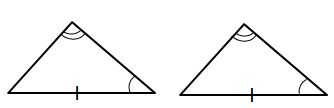#### Question 2 2. You want to try to prove that triangles PQS and PRS are congruent using the HA theorem. You are given that angle P and angle S are congruent and the triangles share a hypotenuse which means the hypotenuses are congruent. What additional information do you need in order to use the HA theorem?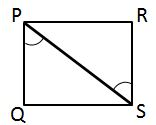#### Question 3 3. If the pictured triangles are congruent, what reason can be given?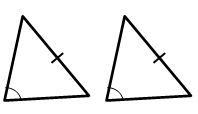#### Question 4 4. If triangle ABD is congruent to triangle CDB, CPCTC explains which of the following statements?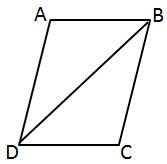#### Question 5 5. In the pictured triangle, XV is an angle bisector. What is the length of XY?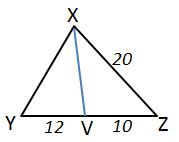### Page 2

#### Question 6 6. Which theorem or postulate can be used to establish congruence with the pictured triangles?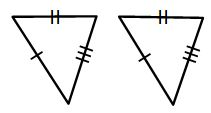#### Question 7 7. What additional information do you need to prove that triangle ABC is congruent to triangle DEF using the HL theorem?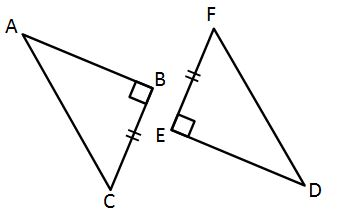#### Question 9 9. You are given that angle B and E are right angles and that the hypotenuses of the triangles are congruent. Which of these is additional information that will help you prove that triangle ABC is congruent to triangle DEF using the HA theorem?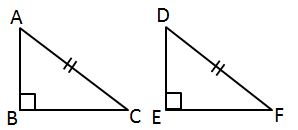#### Question 10 10. If the pictured triangles are congruent, what reason can be given?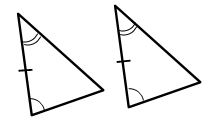### Page 3

#### Question 11 11. Which theorem can be used to establish congruence with the pictured triangles?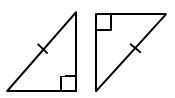#### Question 13 13. In the pictured triangles, what reason can we use to explain that angle QPR is congruent to angle SPT?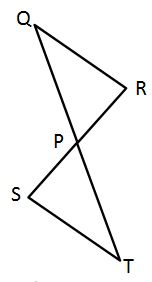#### Question 14 14. What additional information do you need to prove that triangle ACD is congruent to triangle DBA using the HL theorem?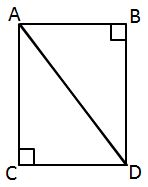#### Question 15 15. Which theorem can be used to establish congruence with the pictured triangles?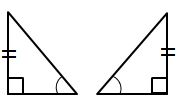### Page 4

#### Question 16 16. Which of the following represents the angle bisector theorem for the pictured triangle?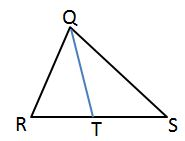#### Question 17 17. In the pictured triangle, MS is an angle bisector. What is the length of SO?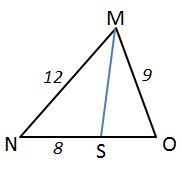#### Question 18 18. How can we explain that the pictured triangles are congruent?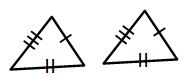#### Question 19 19. In the pictured triangle, FE is an angle bisector. What is the length of GE?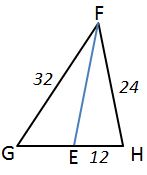### Page 5

#### Question 21 21. Triangle HYV and triangle AYB are similar by the AA similarity theorem. What is the value of x?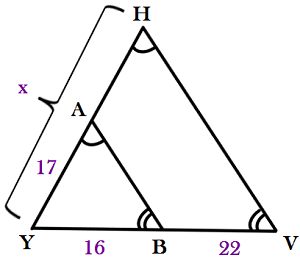#### Question 22 22. Given triangles DAR and KMR. What is the value of y?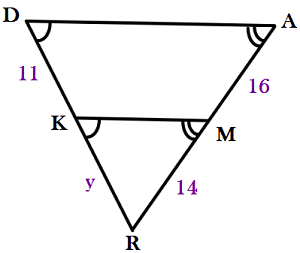#### Question 23 23. In the pictured triangle, AD is an angle bisector. What is the length of AC?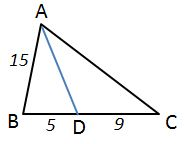#### Question 24 24. Find the measure of XZ: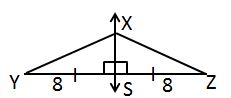#### Question 25 25. If the pictured triangles are congruent, what reason can be given?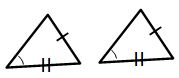### Page 6

#### Question 28 28. Which theorem or postulate can be used to establish congruence with the pictured triangles?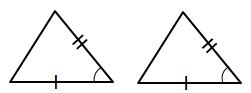#### Question 29 29. If triangle ACB is congruent to triangle YXZ, all of the following are true EXCEPT: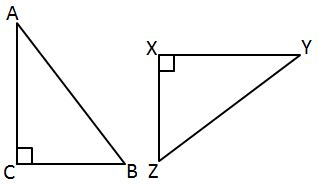#### Question 30 30. If triangle ABC is congruent to triangle RST, all of the following are true EXCEPT: (Remember to look very carefully at the triangles and mark the corresponding sides!)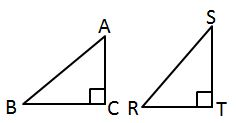#### NMTA Middle Grades Math: Triangle Theorems & Proofs Chapter Exam Instructions

Choose your answers to the questions and click 'Next' to see the next set of questions. You can skip questions if you would like and come back to them later with the yellow "Go To First Skipped Question" button. When you have completed the practice exam, a green submit button will appear. Click it to see your results. Good luck!

Support